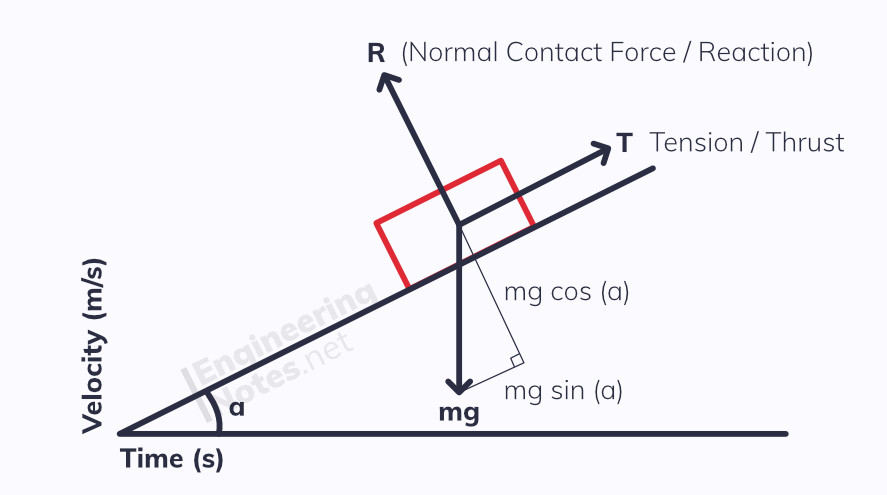#### Notes by Category University Engineering

Electronics*
Mathematics*
Mechanics & Stress Analysis*
Rate these notesNot a fanNot so goodGoodVery goodBrillRate these notes
• A-Level Physics

# Forces & Newton's Laws

First off, it is important to distinguish between mass and weight. Mass is a physical property of an object, dependent on the amount of matter the object is made up of. The weight of an object is the gravitational force acting on its mass. On earth, where g = 9.81, the weight is 9.81 x the mass:

weight = mass x gravity W = mg = 9.81m

## Newton's Laws of Motion

### Newton's 1st Law:

An object will stay at a constant velocity, or motionless, unless it experiences a resultant force.

### Newton's 2nd Law:

The resultant force on an object is directly proportional to the rate of change of momentum of the object, and acts in the same direction.

Therefore, F = Δp / Δt, which is commonly written as F=ma

### Newton's 3rd Law:

For every action, there is an equal but opposite reaction (equal in magnitude and type of force).

## Representing Forces

Weight acts down from an object's centre of gravity. Other forces can act in any direction, and so to avoid confusion it is best to draw a force diagram:The length of arrow should represent the magnitude of the force. Single headed arrows represent forces.

If a system is in equilibrium - meaning there is no net resultant force and it is motionless or at constant velocity - then all the force arrows will make a closed shape. The example above would give a triangle.

So yeh, trigonometry is used a lot...

## Drag & Terminal Velocity

Though not modelled mathematically at A-Level, when objects fall through fluids (liquids & gasses) there is significant drag that slows them down. This means that unless in a vacuum, there is no such thing as free fall as acceleration is not constant.

The magnitude of the drag force depends largely on the shape and texture of the object, the density of the fluid, and the speed of the object - the greater the speed, the greater the drag force, until it equals the weight of the object.

### Terminal Velocity

When this equilibrium is reached, there is no net resultant force on the object anymore, and so (according to Newton's Laws) it stops accelerating. This constant speed is known as terminal velocity.